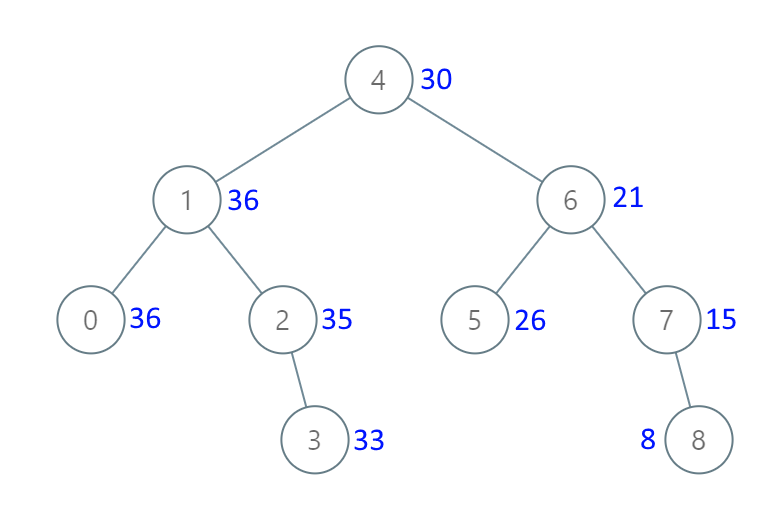Formatted question description: https://leetcode.ca/all/1038.html

# 1038. Binary Search Tree to Greater Sum Tree (Medium)

Given the root of a binary search tree with distinct values, modify it so that every node has a new value equal to the sum of the values of the original tree that are greater than or equal to node.val.

As a reminder, a binary search tree is a tree that satisfies these constraints:

• The left subtree of a node contains only nodes with keys less than the node's key.
• The right subtree of a node contains only nodes with keys greater than the node's key.
• Both the left and right subtrees must also be binary search trees.

Example 1:Input: [4,1,6,0,2,5,7,null,null,null,3,null,null,null,8]
Output: [30,36,21,36,35,26,15,null,null,null,33,null,null,null,8]


Note:

1. The number of nodes in the tree is between 1 and 100.
2. Each node will have value between 0 and 100.
3. The given tree is a binary search tree.

Companies:
Amazon

Related Topics:
Binary Search Tree

## Solution 1.

// OJ: https://leetcode.com/problems/binary-search-tree-to-greater-sum-tree/

// Time: O(N)
// Space: O(N)
class Solution {
private:
int sum = 0;
public:
TreeNode* bstToGst(TreeNode* root) {
if (!root) return NULL;
bstToGst(root->right);
root->val = (sum += root->val);
bstToGst(root->left);
return root;
}
};


Java

/**
* Definition for a binary tree node.
* public class TreeNode {
*     int val;
*     TreeNode left;
*     TreeNode right;
*     TreeNode(int x) { val = x; }
* }
*/
class Solution {
public TreeNode bstToGst(TreeNode root) {
if (root == null)
return root;
int prevVal = 0;
Stack<TreeNode> stack = new Stack<TreeNode>();
TreeNode node = root;
while (!stack.isEmpty() || node != null) {
while (node != null) {
stack.push(node);
node = node.right;
}
TreeNode visitNode = stack.pop();
visitNode.val += prevVal;
prevVal = visitNode.val;
node = visitNode.left;
}
return root;
}
}